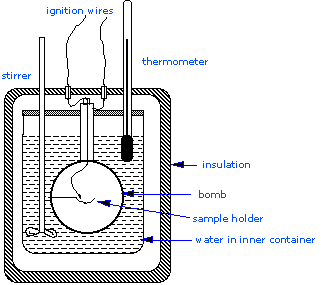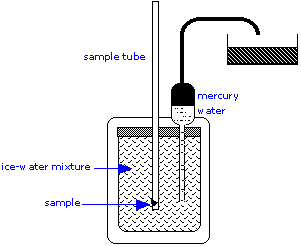# 14.5: Calorimetry

•• Contributed by Stephen Lower
• Professor Emeritus (Chemistry) at Simon Fraser University

Learning Objectives

Make sure you thoroughly understand the following essential concept:

• Describe a simple calorimeter and explain how it is employed and how its heat capacity is determined.

Constant Volume Calorimetry, also know as bomb calorimetry, is used to measure the heat of a reaction while holding volume constant and resisting large amounts of pressure. Although these two aspects of bomb calorimetry make for accurate results, they also contribute to the difficulty of bomb calorimetry. In this module, the basic assembly of a bomb calorimeter will be addressed, as well as how bomb calorimetry relates to the heat of reaction and heat capacity and the calculations involved in regards to these two topics.

## Introduction

Calorimetry is used to measure quantities of heat, and can be used to determine the heat of a reaction through experiments. Usually a coffee-cup calorimeter is used since it is simpler than a bomb calorimeter, but to measure the heat evolved in a combustion reaction, constant volume or bomb calorimetry is ideal. A constant volume calorimeter is also more accurate than a coffee-cup calorimeter, but it is more difficult to use since it requires a well-built reaction container that is able to withstand large amounts of pressure changes that happen in many chemical reactions.Figure $$\PageIndex{1}$$: A simplified bomb calorimeter.

Most serious calorimetry carried out in research laboratories involves the determination of heats of combustion $$\Delta H_{combustion}$$, since these are essential to the determination of standard enthalpies of formation of the thousands of new compounds that are prepared and characterized each month. In a constant volume calorimeter, the system is sealed or isolated from its surroundings, which accounts for why its volume is fixed and there is no volume-pressure work done. A bomb calorimeter structure consists of the following:

• Steel bomb which contains the reactants
• Water bath in which the bomb is submerged
• Thermometer
• A motorized stirrer
• Wire for ignition

Since the process takes place at constant volume, the reaction vessel must be constructed to withstand the high pressure resulting from the combustion process, which amounts to a confined explosion. The vessel is usually called a “bomb”, and the technique is known as bomb calorimetry. The reaction is initiated by discharging a capacitor through a thin wire which ignites the mixture.

Another consequence of the constant-volume condition is that the heat released corresponds to $$q_v$$, and thus to the internal energy change $$ΔU$$ rather than to $$ΔH$$. The enthalpy change is calculated according to the formula

$ΔH = q_v + Δn_gRT$

where $$Δn_g$$  is the change in the number of moles of gases in the reaction.

Example $$\PageIndex{1}$$: Combustion of Biphenyl

A sample of biphenyl ($$\ce{(C6H5)2}$$) weighing 0.526 g was ignited in a bomb calorimeter initially at 25°C, producing a temperature rise of 1.91 K. In a separate calibration experiment, a sample of benzoic acid ($$\ce{C6H5COOH}$$) weighing 0.825 g was ignited under identical conditions and produced a temperature rise of 1.94 K. For benzoic acid, the heat of combustion at constant pressure is known to be 3226 kJ mol–1 (that is, ΔU = –3226 kJ mol–1.) Use this information to determine the standard enthalpy of combustion of biphenyl.

Solution

Begin by working out the calorimeter constant:

• Moles of benzoic acid:

$\dfrac{0.825 g}{122.1 \;g/mol} = 0.00676\; mol \nonumber$

• Heat released to calorimeter:

$(0.00676\; mol) \times (3226\; kJ/mol) = 21.80\; kJ \nonumber$

• Calorimeter constant:

$\dfrac{21.80\; kJ}{1.94\; K} = 11.24\; kJ/K \nonumber$

Now determine $$ΔU_{combustion}$$ of the biphenyl ("BP"):

• moles of biphenyl:

$\dfrac{0.526\; g}{154.12\; g/mol} = 0.00341 \; mol \nonumber$

• heat released to calorimeter:

$(1.91\; K) \times (11.24\; kJ/K) = 21.46\; kJ \nonumber$

• heat released per mole of biphenyl:

$\dfrac{21.46\; kJ}{0.00341\; mol} = 6,293\; kJ/mol \nonumber$

$ΔU_{combustion} (BP) = –6,293\; kJ/mol \nonumber$

This is the heat change at constant volume, $$q_v$$; the negative sign indicates that the reaction is exothermic, as all combustion reactions are.

From the balanced reaction equation

$\ce{(C6H5)2(s) + 29/2 O2(g) \rightarrow 12 CO2(g) + 5 H2O(l)} \nonumber$

we can calculate the change in the moles of gasses for this reaction

$Δn_g = 12 - \frac{29}{2} = \frac{-5}{2} \nonumber$

Thus the volume of the system decreases when the reaction takes place. Converting to $$ΔH$$, we can write the following equation. Additionally, recall that at constant volume, $$ΔU = q_V$$.

\begin{align*} ΔH &= q_V + Δn_gRT \\[4pt] &= ΔU -\left( \dfrac{5}{2}\right) (8.314\; J\; mol^{-1}\; K^{-1}) (298 \;K) \\[4pt] &= (-6,293 \; kJ/mol)–(6,194\; J/mol) \\[4pt] &= (-6,293-6.2)\;kJ/mol \\[4pt] &= -6299 \; kJ/mol \end{align*}

A common mistake here is to forget that the subtracted term is in J, not kJ. Note that the additional 6.2 kJ in $$ΔH$$ compared to $$ΔU$$ reflects the work that the surroundings do on the system as the volume of gases decreases according to the reaction equation.

### Determining the Heat of Reaction

The amount of heat that the system gives up to its surroundings so that it can return to its initial temperature is the heat of reaction. The heat of reaction is just the negative of the thermal energy gained by the calorimeter and its contents ($$q_{calorimeter}$$) through the combustion reaction.

$q_{rxn} = -q_{calorimeter} \label{2A}$

where

$q_{calorimeter} = q_{bomb} + q_{water} \label{3A}$

If the constant volume calorimeter is set up the same way as before, (same steel bomb, same amount of water, etc.) then the heat capacity of the calorimeter can be measured using the following formula:

$q_{calorimeter} = \text{( heat capacity of calorimeter)} \times \Delta{T} \label{4A}$

Heat capacity is defined as the amount of heat needed to increase the temperature of the entire calorimeter by 1 °C. The equation above can also be used to calculate $$q_{rxn}$$ from $$q_{calorimeter}$$ calculated by Equation \ref{2A}. The heat capacity of the calorimeter can be determined by conducting an experiment.

Example $$\PageIndex{4}$$: Heat of Combustion

1.150 g of sucrose goes through combustion in a bomb calorimeter. If the temperature rose from 23.42 °C to 27.64 °C and the heat capacity of the calorimeter is 4.90 kJ/°C, then determine the heat of combustion of sucrose, $$\ce{C12H22O11}$$ (in kJ per mole of $$\ce{C12H22O11}$$).

Solution

Given:

• mass of $$C_{12}H_{22}O_{11}$$: 1.150 g
• $$T_{initial}$$: 23.42°C
• $$T_{final}$$:27.64°C
• Heat Capacity of Calorimeter: 4.90 kJ/°C

Using Equation \ref{4A} to calculate $$q_{calorimeter}$$:

\begin{align*} q_{calorimeter} &= (4.90\; kJ/°C) \times (27.64 - 23.42)°C \\[4pt] &= (4.90 \times 4.22) \;kJ = 20.7\; kJ \end{align*}

Plug into Equation \ref{2A}:

\begin{align*} q_{rxn} &= -q_{calorimeter} \\[4pt] &= -20.7 \; kJ \; \end{align*}

But the question asks for kJ/mol $$\ce{C12H22O11}$$, so this needs to be converted:

\begin{align*}q_{rxn} &= \dfrac{-20.7 \; kJ}{1.150 \; g \; C_{12}H_{22}O_{11}} \\[4pt] &= \dfrac{-18.0 \; kJ}{g\; C_{12}H_{22}O_{11}} \end{align*}

Convert to per Mole $$\ce{C12H22O11}$$:

\begin{align*}q_{rxn} &= \dfrac{-18.0 \; kJ}{\cancel{g \; \ce{C12H22O11}}} \times \dfrac{342.3 \; \cancel{ g \; \ce{C12H22O11}}}{1 \; mol \; \ce{C12H22O11}} \\[4pt] &= \dfrac{-6.16 \times 10^3 \; kJ \;}{mol \; \ce{C12H22O11}} \end{align*}

"Ice Calorimeter"

Although calorimetry is simple in principle, its practice is a highly exacting art, especially when applied to processes that take place slowly or involve very small heat changes, such as the germination of seeds. Calorimeters can be as simple as a foam plastic coffee cup, which is often used in student laboratories. Research-grade calorimeters, able to detect minute temperature changes, are more likely to occupy table tops, or even entire rooms:The ice calorimeter is an important tool for measuring the heat capacities of liquids and solids, as well as the heats of certain reactions. This simple yet ingenious apparatus is essentially a device for measuring the change in volume due to melting of ice. To measure a heat capacity, a warm sample is placed in the inner compartment, which is surrounded by a mixture of ice and water.

The heat withdrawn from the sample as it cools causes some of the ice to melt. Since ice is less dense than water, the volume of water in the insulated chamber decreases. This causes an equivalent volume of mercury to be sucked into the inner reservoir from the outside container. The loss in weight of this container gives the decrease in volume of the water, and thus the mass of ice melted. This, combined with the heat of fusion of ice, gives the quantity of heat lost by the sample as it cools to 0°C.